# Heat Transfer by Natural Convection (Part - 2) Chemical Engineering Notes | EduRev

## Chemical Engineering : Heat Transfer by Natural Convection (Part - 2) Chemical Engineering Notes | EduRev

The document Heat Transfer by Natural Convection (Part - 2) Chemical Engineering Notes | EduRev is a part of the Chemical Engineering Course Heat Transfer for Engg..
All you need of Chemical Engineering at this link: Chemical Engineering

5.2 Empirical relations for natural-convective heat transfer

5.2.1 Natural convection around a flat vertical surface

Churchill and Chu provided the correlation for average heat transfer coefficient for natural convection for different ranges of Rayleigh number.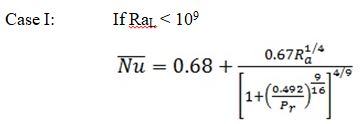(5.7)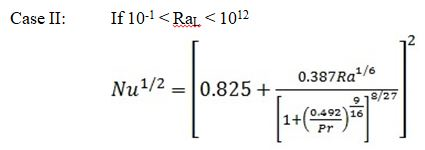(5.8)

It should be noted that the eq. 5.7 and 5.8 are also applicable for an inclined surface upto less than inclination from the vertical plane.

The above relations can be used for the vertical cylinder if the boundary layer thickness is quite small as compared to the diameter of the cylinder. The criteria to use the above relation for vertical cylinder is,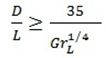(5.9)

where,  is the diameter and  is the height of the cylinder.

5.2.2 Natural convection around a horizontal cylinder

Churchill and Chu has provided the following expression for natural-convective heat transfer.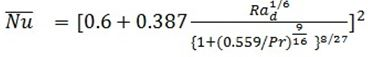(5.10)

Condition of applicability of the eq.5.10:

5.2.3 Natural convection around a horizontal flat surface

In the previous case of vertical flat surface, the principal body dimension was in-line with the gravity (i.e. vertical). Therefore, the flow produced by the free convection was parallel to the surface regardless of whether the surface was hotter or cooler compared to the bulk fluid around. However, in case of horizontal flat plate the flow pattern will be different and shown in fig. 5.3.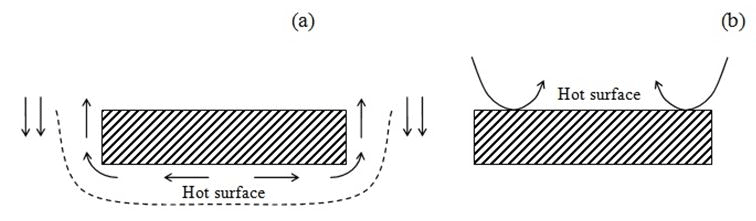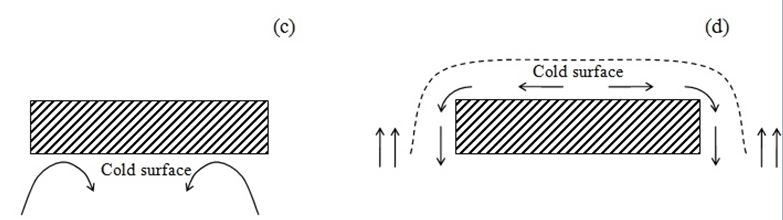Fig. 5.3: A representative flow pattern (natural convection) for (a) hot surface down, (b) hot surface up, (c) cold surface down, and (d) cold surface up

Thus from fig. 5.3 it is understood that there are in fact two cases (i) when the heated plate facing up or cooled plate facing down, and (ii) heated plate facing down or cooled plate facing up.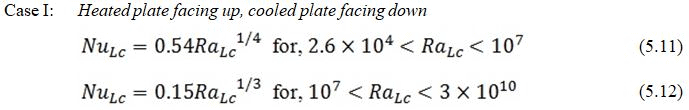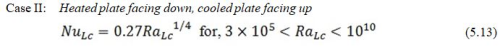where, Lc is characteristic length defined as below.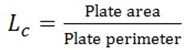5.2.4 Natural convection around sphere

Churchill proposed,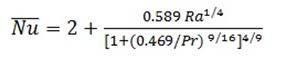(5.14)

Condition for applicability: Pr ≥ 0.7; Ra ≤ 1011

5.2.5 Natural convection in enclosure

It is another class of problems for which there are many cases and their corresponding correlations are also available in the literature. Here two cases will be discussed, (i) in which a fluid is contained between two vertical plates separated by a distance d, (ii) the other where the fluid is in an annulus formed by two concentric horizontal cylinders.

In the case first, the plates are at different temperature, T1 and T2. Heat transfer will be from higher temperature (T1) to lower temperature (T2) through the fluid.

The corresponding Grashof number will be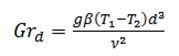McGregor and Emery proposed the following correction for free convection heat transfer in a vertical rectangular enclosure, where the vertical walls are heated or cooled and the horizontal surfaces many be assumed adiabatic,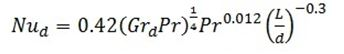(5.15)

Applicability conditions for the above equation are,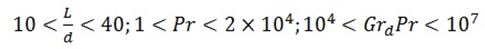or,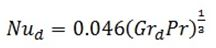(5.16)

Applicability conditions are,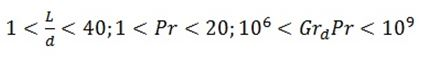Here L/d is known as the aspect ratio.

At steady state condition, the heat flux (qx) is equal thus,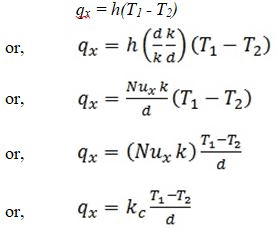where, kc(= Nuxk) is known as the apparent thermal conductivity.

In the second case the heat transfer is involved in the enclosure formed by two concentric cylinders in horizontal position, the correlation given by Raithby and Holland,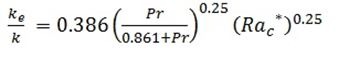(5.17)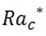is the modified Rayleigh number given by,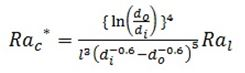where, di and d0 are the outer and inner diameter of the inner and outer cylinders, respectively. The enclosure characteristic length l is defined as (d0 - di).

The applicability of the eq. 5.17 is 102> 107.

It should be noted that the rate of heat flow by natural convection per unit length is same as that through the annular cylindrical region having effective thermal conductivity ke for the case,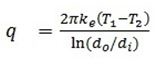where, T1 and T2 are the temperatures of the inner and outer cylindrical walls, respectively.

Offer running on EduRev: Apply code STAYHOME200 to get INR 200 off on our premium plan EduRev Infinity!

## Heat Transfer for Engg.

46 videos|60 docs|59 tests

,

,

,

,

,

,

,

,

,

,

,

,

,

,

,

,

,

,

,

,

,

;